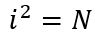# Problem 50152. Number Puzzle - 037

Give an example of a number i where i>1000 that satisfies the following conditions:and N does not have the digit "7" in it but the sum of the digits of N is equal to 7^2 or 49.

### Solution Stats

62.22% Correct | 37.78% Incorrect
Last Solution submitted on Nov 20, 2023

### Community Treasure Hunt

Find the treasures in MATLAB Central and discover how the community can help you!

Start Hunting!